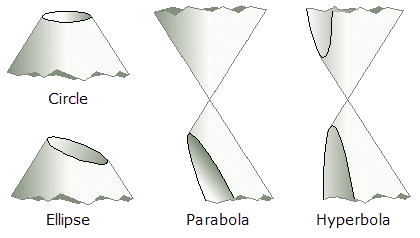# ConicCurve

Conic

The Conic command draws a conic section curve based on a start, end, and apex point. The default is to first pick a start point, then an end point, and then an apex point.#### Default steps

1. Pick the start of the conic curve.
2. Pick the end of the curve.
3. Pick the apex of the conic curve.
This location partially defines the plane for the conic.
4. Pick a location for the conic to pass through to define its curvature.
Or, type a the rho value (a number greater than 0 and less than 1), and press Enter.
Command-line options

Apex

Lets you specify the apex point before you pick the endpoint of the conic curve.

#### Apex steps

1. Pick the start of the conic curve.
2. Pick the apex ("top" control point) of the conic curve.
3. Pick the end of the conic curve.

Tangent

Lets you select a curve for the conic curve to be tangent to for the start or end of the conic.

#### Steps

1. Select a curve for the conic curve to be tangent to.
2. Drag the tangent indicator to the desired location and pick the location for the start or end of the conic curve.

Perpendicular

Lets you select a curve for the conic to be perpendicular to for the start or end of the conic.

#### Steps

1. Select a curve for the conic curve to be perpendicular to.
2. Drag the perpendicular indicator to the desired location and pick the location for the start or end of the conic curve.

Type subcrv to select part of a curve as input.

#####Hyperbola

Draw a hyperbolic curve from focus points, vertices, or coefficient.

#####Parabola

Draw a parabolic curve from focus and vertex or endpoint.

Rhinoceros 7 © 2010-2021 Robert McNeel & Associates. 20-Oct-2021